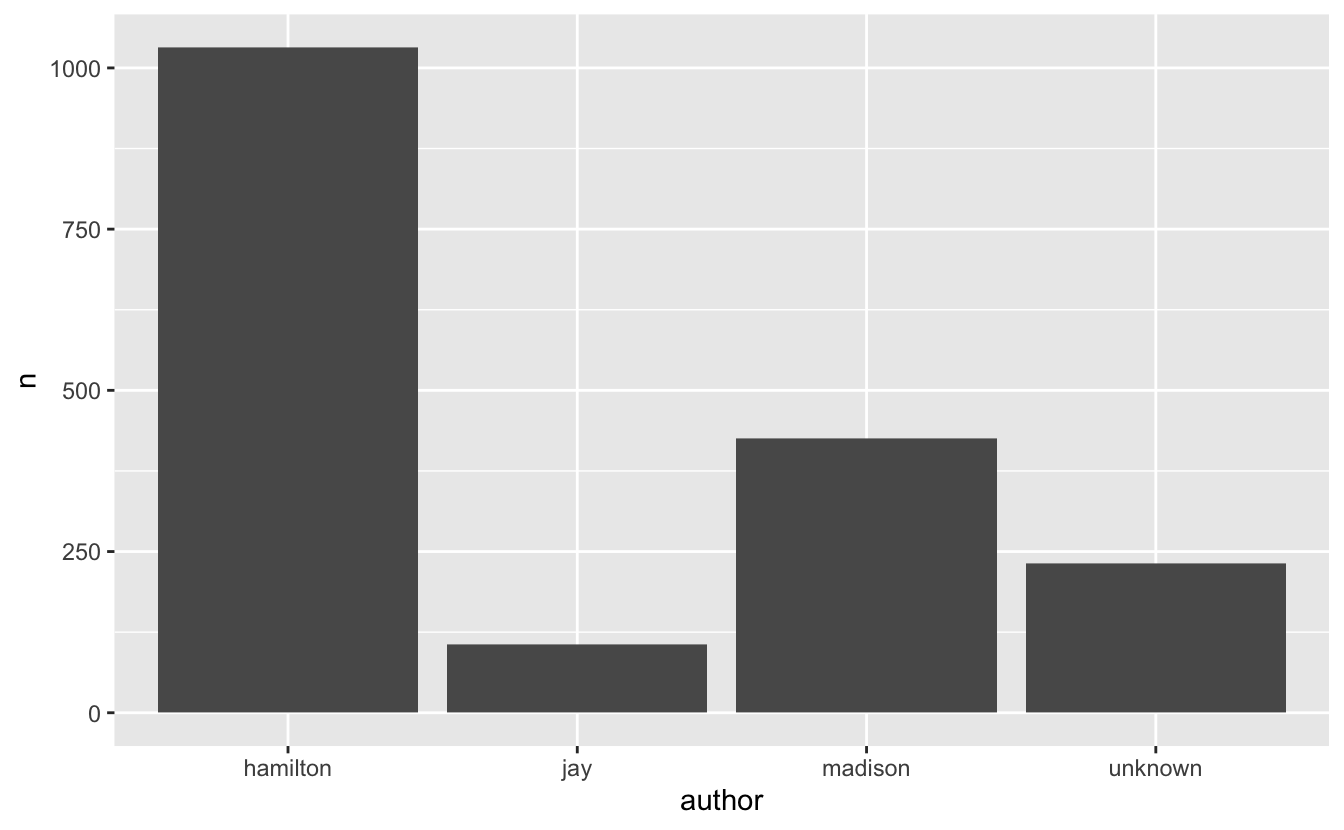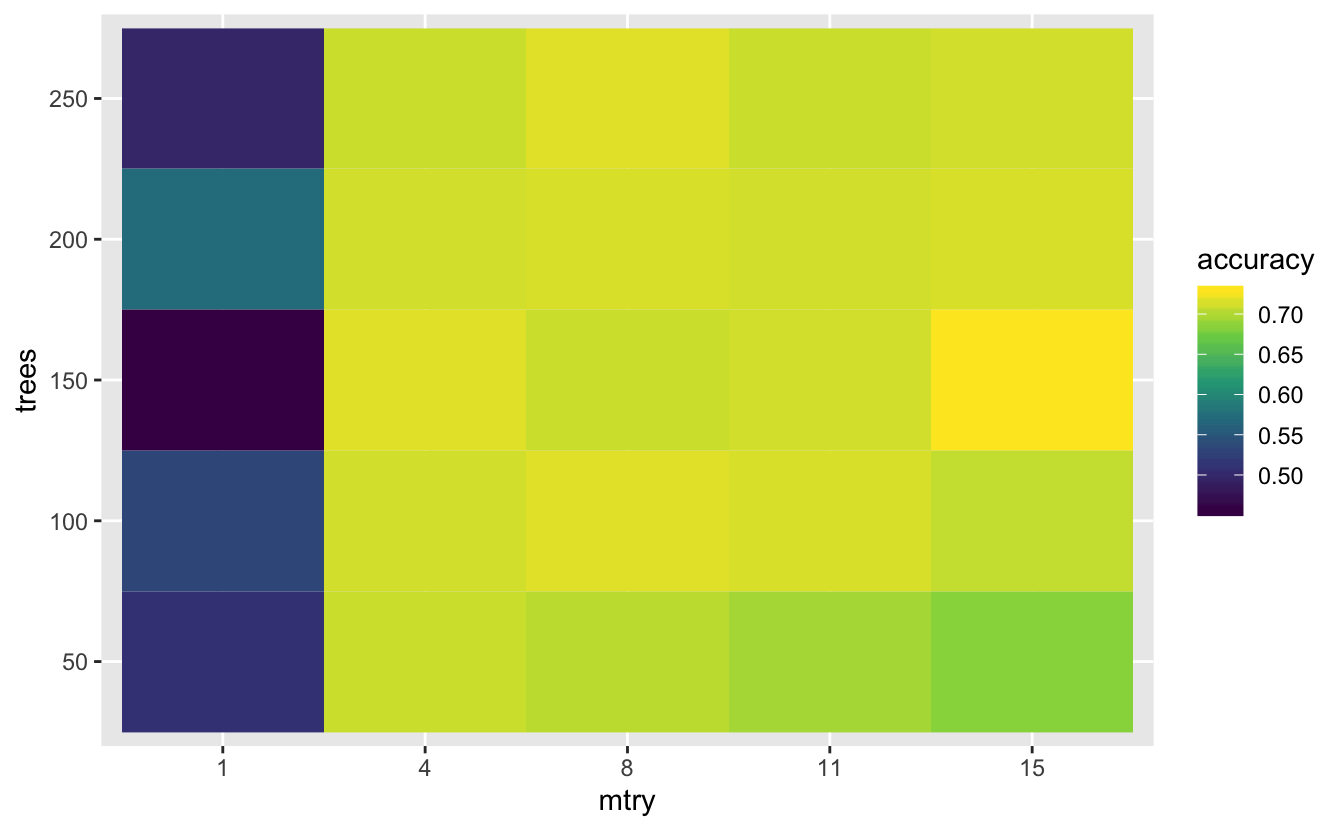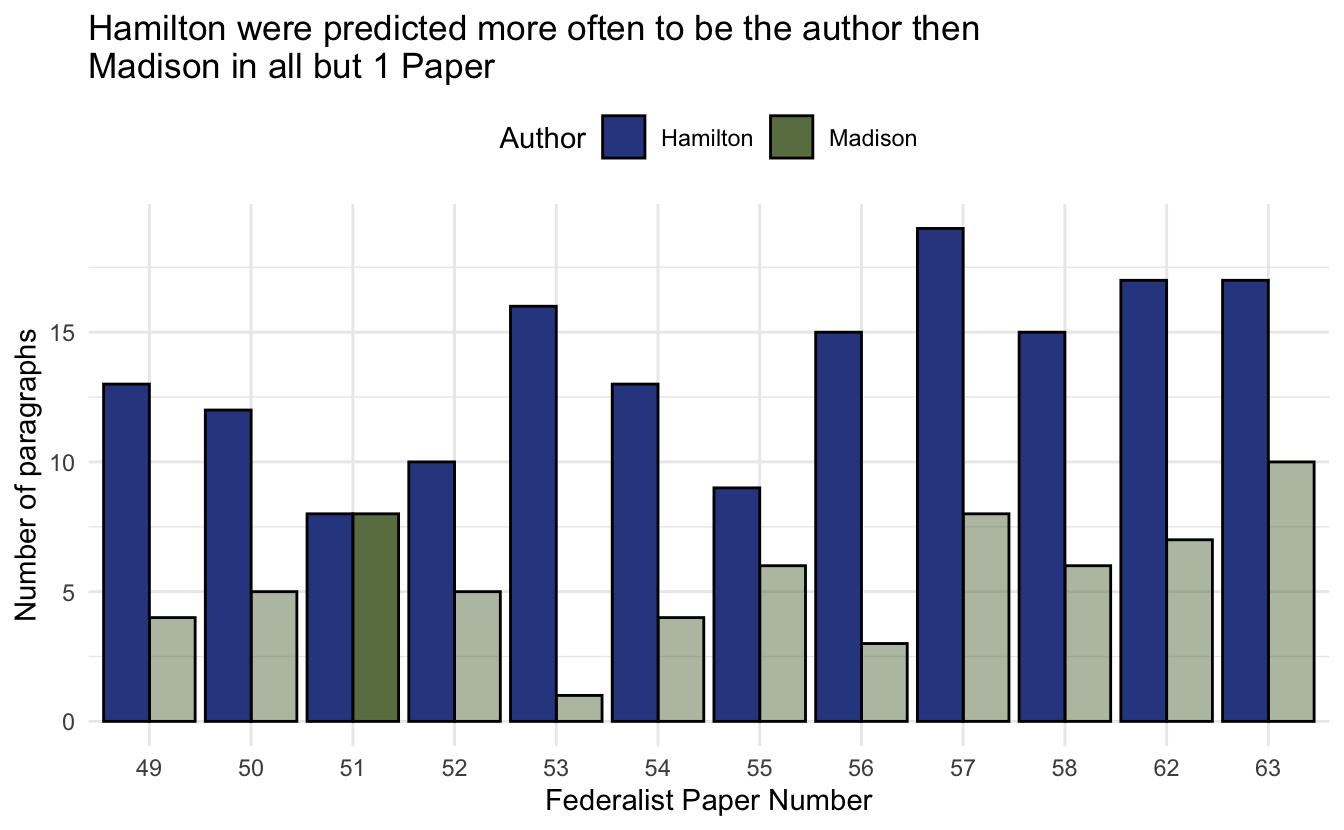# Authorship classification with tidymodels and textrecipes

In this post we will revisit on of my earlier blogposts where I tried to use tidytext and glmnet to predict the authorship of the anonymous Federalist Papers. If you want more information regarding the data, please read the old post. In the post we will try to achieve the same goal, but use the tidymodels framework.

``library(tidymodels) # Modeling framework``
``````## Registered S3 method overwritten by 'xts':
##   method     from
##   as.zoo.xts zoo``````
``## ── Attaching packages ───────────────────────────── tidymodels 0.0.2 ──``
``````## ✔ broom     0.5.2       ✔ purrr     0.3.2
## ✔ dials     0.0.2       ✔ recipes   0.1.6
## ✔ dplyr     0.8.3       ✔ rsample   0.0.5
## ✔ ggplot2   3.2.0       ✔ tibble    2.1.3
## ✔ infer     0.4.0.1     ✔ yardstick 0.0.3
## ✔ parsnip   0.0.3.1``````
``````## ── Conflicts ──────────────────────────────── tidymodels_conflicts() ──
## ✖ dplyr::filter()  masks stats::filter()
## ✖ dplyr::lag()     masks stats::lag()
## ✖ recipes::step()  masks stats::step()``````
``````library(textrecipes) # extension to preprocessing engine to handle text
library(stringr) # String modification``````
``````##
## Attaching package: 'stringr'``````
``````## The following object is masked from 'package:recipes':
##
##     fixed``````
``````library(gutenbergr) # Portal to download the Federalist Papers
library(tokenizers) # Tokenization engine
library(furrr) # to be able to fit the models in parallel``````
``## Loading required package: future``

## Fetching the Data

The text is provided from the Gutenberg Project. A simple search reveals that the Federalist Papers have the id of 1404.

``papers <- gutenberg_download(1404)``
``## Determining mirror for Project Gutenberg from http://www.gutenberg.org/robot/harvest``
``## Using mirror http://aleph.gutenberg.org``
``papers``
``````## # A tibble: 19,359 x 2
##    gutenberg_id text
##           <int> <chr>
##  1         1404 THE FEDERALIST PAPERS
##  2         1404 ""
##  3         1404 By Alexander Hamilton, John Jay, and James Madison
##  4         1404 ""
##  5         1404 ""
##  6         1404 ""
##  7         1404 ""
##  8         1404 FEDERALIST No. 1
##  9         1404 ""
## 10         1404 General Introduction
## # … with 19,349 more rows``````

## shaping data

This is the first we will deviate from the original post in that we will divide the text into paragraphs instead of sentences as we did in the last post. Hopefully this will strike a good balance between size of each observation and the number of observations.

In the following pipe we: - `pull()` out the text vector - paste together the strings with `\n` to denote line-breaks - tokenize into paragraphs - put it in a tibble - create a variable `no` to denote which paper the paragraph is in - add `author` variable to denote author - remove preamble text

``````# attribution numbers
hamilton <- c(1, 6:9, 11:13, 15:17, 21:36, 59:61, 65:85)
madison <- c(10, 14, 18:20, 37:48)
jay <- c(2:5, 64)
unknown <- c(49:58, 62:63)

papers_paragraphs <- papers %>%
pull(text) %>%
str_c(collapse = "\n") %>%
tokenize_paragraphs() %>%
unlist() %>%
tibble(text = .) %>%
mutate(no = cumsum(str_detect(text, regex("FEDERALIST No",
ignore_case = TRUE)))) %>%
mutate(author = case_when(no %in% hamilton ~ "hamilton",
no %in% jay ~ "jay",
no %in% unknown ~ "unknown")) %>%
filter(no > 0)``````

## Class Balance

There is quite a bit inbalence between the classes. For the remaining of the analysis will we exclude all the papers written by `Jay`, partly because it is a small class, but more importantly because he isn’t suspected to be the mystery author.

``````papers_paragraphs %>%
count(author) %>%
ggplot(aes(author, n)) +
geom_col()``````It is wroth remembering that we don’t have the true answer, much more like in real world problems.

## Splitting the Data

Here we will use the `rsample` package to split the data into a testing, validation and training dataset. We will let the testing dataset be all the paragraphs where `author == "unknown"` and the training and validation datasets being the paragraphs written by Hamilton and Madison. `intial_split()` will insure that each dataset with have the same proportions with respect to the `author` column.

``````data_split <- papers_paragraphs %>%
filter(author %in% c("hamilton", "madison")) %>%
initial_split(strata = author)

training_data <- training(data_split)

validation_data <- testing(data_split)

testing_data <- papers_paragraphs %>%
filter(author == "unknown")``````

## specifying data preprocessing

We will go with a rather simple preprocessing. start by specifying a recipe where `author` is to be predicted, and we only want to use the `text` data. Here we make sure to use the training dataset. We then

and finally prep the recipe.

``````rec <- recipe(author ~ text, data = training_data) %>%
step_tokenize(text, token = "ngrams", options = list(n = 3)) %>%
step_tokenfilter(text, max_tokens = 250) %>%
step_tfidf(text) %>%
step_upsample(author) %>%
prep()``````

## Apply Preprocessing

Now we apply the prepped recipe to get back the processed datasets. Note that I have used shorter names for processed datasets (`train_data` vs `training_data`).

``````train_data <- juice(rec)
val_data <- bake(rec, new_data = validation_data)
test_data <- bake(rec, new_data = testing_data)``````

## Fitting the Models

This time I’m going to try to run some (random forests)[https://en.wikipedia.org/wiki/Random_forest]. And that would be fairly easy to use. First we specify the the model type (`rand_forest`) the type (`classification`) and the engine (`randomForest`). Next we fit the model to the training dataset, predict it on the validation datasets, add the true value and calculate the accuracy

``````rand_forest("classification") %>%
set_engine("randomForest") %>%
fit(author ~ ., data = train_data) %>%
predict(new_data = val_data) %>%
mutate(truth = val_data\$author) %>%
accuracy(truth, .pred_class)``````
``````## # A tibble: 1 x 3
##   .metric  .estimator .estimate
##   <chr>    <chr>          <dbl>
## 1 accuracy binary         0.712``````

However we want to try some different hyper-parameter values to make sure we are using the best we can. The `dials` allows us to do hyper-parameter searching in a fairly easy way. First we will create a parameter_grid, where we will vary the number of trees in our forest (`trees`) and the number of predictors to be randomly sampled. We give it some reasonable ranges, and say that we want 5 levels for each parameter, resulting in `5 * 5 = 25` parameter pairs.

``````param_grid <- grid_regular(range_set(trees, c(50, 250)),
range_set(mtry, c(1, 15)), levels = 5)``````

Next we create a model specification where we use `varying()` to denote that these parameters are to be varying. Then we `merge()` the model specification into the parameter grid such that we have a tibble of model specifications

``````rf_spec <- rand_forest("classification", mtry = varying(), trees = varying()) %>%
set_engine("randomForest")

param_grid <- param_grid %>%
mutate(specs = merge(., rf_spec))

param_grid``````
``````## # A tibble: 25 x 3
##    trees  mtry specs
##  * <int> <int> <list>
##  1    50     1 <spec[+]>
##  2   100     1 <spec[+]>
##  3   150     1 <spec[+]>
##  4   200     1 <spec[+]>
##  5   250     1 <spec[+]>
##  6    50     4 <spec[+]>
##  7   100     4 <spec[+]>
##  8   150     4 <spec[+]>
##  9   200     4 <spec[+]>
## 10   250     4 <spec[+]>
## # … with 15 more rows``````

Next we want to iterate through the model specification. We will here create a function that will take a model specification, fit it to the training data, predict according to the validation data, calculate the accuracy and return it as a single number. Create this function makes it so we can use `map()` over all the model specifications.

``````fit_one_spec <- function(model) {
model %>%
fit(author ~ ., data = train_data) %>%
predict(new_data = val_data) %>%
mutate(truth = val_data\$author) %>%
accuracy(truth, .pred_class) %>%
pull(.estimate)
}``````

While this is a fairly small dataset, I’ll showcase how we can parallize the calculations. Since we have a framework where are we `map()`’ing over the specification it is a obvious case for the `furrr` package. (if you don’t want or isn’t able to to run your models on multiple cores, simply delete `plan(multicore)` and turn `future_map_dbl()` to `map_dbl()`).

``````plan(multicore)
final <- param_grid %>%
mutate(accuracy = future_map_dbl(specs, fit_one_spec))``````

Now we can try to visualize the optimal hyper-parameters

``````final %>%
mutate_at(vars(trees:mtry), factor) %>%
ggplot(aes(mtry, trees, fill = accuracy)) +
geom_tile() +
scale_fill_viridis_c()``````and we clearly see that only having 1 predictor to split with it sub-optimal, but otherwise having a low number of predictors are to be preferred. We can use `arrange()` to look at the top parameter pairs

``````arrange(final, desc(accuracy)) %>%
slice(1:5)``````
``````## # A tibble: 5 x 4
##   trees  mtry specs     accuracy
## * <int> <int> <list>       <dbl>
## 1   150    15 <spec[+]>    0.728
## 2   150     4 <spec[+]>    0.717
## 3   100     8 <spec[+]>    0.717
## 4   250     8 <spec[+]>    0.717
## 5   200     8 <spec[+]>    0.714``````

and we pick `trees == 100` and `mtry == 4` as our hyper-parameters. And we use these to fit our final model

``````final_model <- rf_spec %>%
update(trees = 100, mtry = 4) %>%
fit(author ~ ., data = train_data)``````

## Predicting the unknown papers

Lastly we predict on the unknown papers.

``````final_predict <- testing_data %>%
bind_cols(predict(final_model, new_data = test_data)) ``````

We can’t calculate an accuracy or any other metric, as we don’t know the true value. However we can see how the the different paragraphs have been classified within each paper.

``````final_predict %>%
count(no, .pred_class) %>%
mutate(no = factor(no)) %>%
group_by(no) %>%
mutate(highest = n == max(n))``````
``````## # A tibble: 24 x 4
## # Groups:   no 
##    no    .pred_class     n highest
##    <fct> <fct>       <int> <lgl>
##  1 49    hamilton       13 TRUE
##  2 49    madison         4 FALSE
##  3 50    hamilton       12 TRUE
##  4 50    madison         5 FALSE
##  5 51    hamilton        8 TRUE
##  6 51    madison         8 TRUE
##  7 52    hamilton       10 TRUE
##  8 52    madison         5 FALSE
##  9 53    hamilton       16 TRUE
## 10 53    madison         1 FALSE
## # … with 14 more rows``````

Now we can visualize the results, and it looks like from this limited analysis that Hamilton is the author of mysterious papers.

``````final_predict %>%
count(no, .pred_class) %>%
mutate(no = factor(no),
.pred_class = factor(.pred_class,
levels = c("hamilton", "madison"),
labels = c("Hamilton", "Madison"))) %>%
group_by(no) %>%
mutate(highest = n == max(n)) %>%
ggplot(aes(no, n, fill = .pred_class, alpha = highest)) +
scale_alpha_ordinal(range = c(0.5, 1)) +
geom_col(position = "dodge", color = "black") +
theme_minimal() +
scale_fill_manual(values = c("#304890", "#6A7E50")) +
guides(alpha = "none") +
theme(legend.position = "top") +
labs(x = "Federalist Paper Number",
y = "Number of paragraphs",
fill = "Author",
title = "Hamilton were predicted more often to be the author then\nMadison in all but 1 Paper")``````## Conclusion

In this post we have touched a lot of different topics; tidymodels, text preprocessing, parallel computing etc. Since we have covered so many topics have left each section not covered it a lot of detail. In a more proper analysis you would want to try some different models and different ways to do the preprocessing.